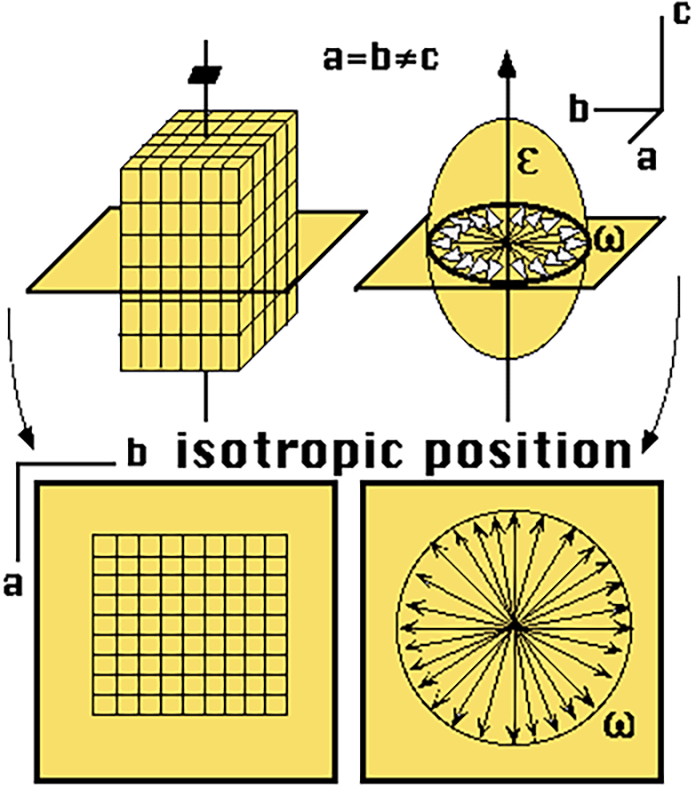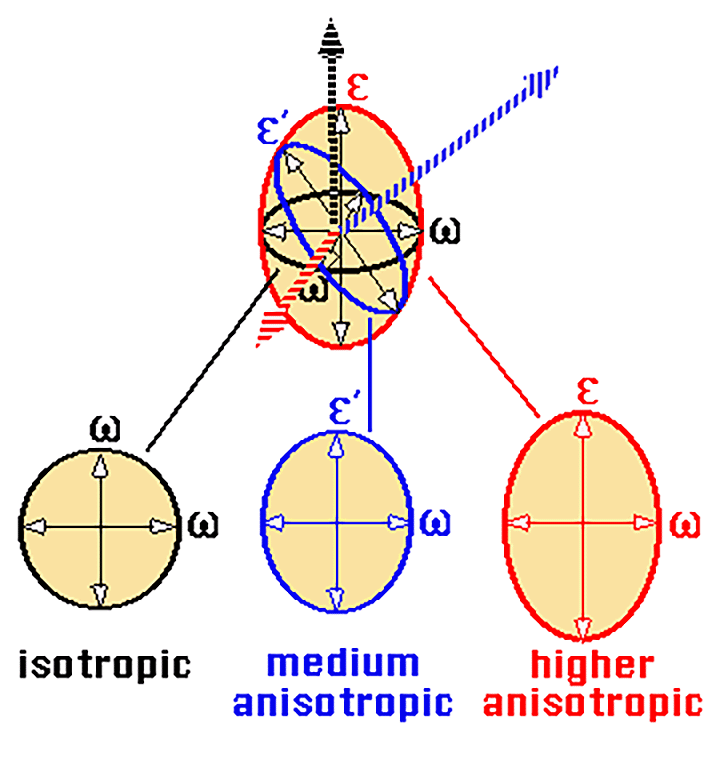Uniaxial indicatrix

In uniaxial crystals, the optic indicatrix is an ellipsoid of revolution (with two main axes and a circular horizontal plane).

Position of isotropy

The direction of the optic axis (vertical axis or "c" axis) coincides with the direction of the axis with greatest crystalline symmetry (quaternary axis).

The waves which are propagated according to the optic axis vibrate in any direction of the horizontal plane.

.General position

When light arrives from another direction, the mineral behaves anisotropically and the refractive index will vary according to this direction.

In the previous position, we considered the position of isotropy, which would correspond to the figure with vertical wave propagation (according to the optic axis shown as the broken black arrow). These waves vibrate in any direction of the horizontal plane, with a single refractive index n omega (for simplicity the diagram, omega has been represented instead of n omega).

If the propagation of the waves now follows a horizontal path, e.g. the one marked by the red arrow, the waves which vibrated following this path would vibrate in any direction contained in the vertical plane of the diagram. The values of their refractive indices would present a an extreme variation between the minimum index n omega and the maximum value n epsilon. For some inclined waves, such as the one represented by the blue arrow, the values of their refractive indices would oscillate between that of n epsilon prime and that of n omega.Para conocer el valor de los índices de refracción de las dos ondas que se propagan según una determinada dirección (recordemos, vibrando perpendicularmente, entre sí y a la de la propagación) en un cristal uniáxico basta trazar un plano perpendicular a esta dirección de propagación que corte a la indicatriz óptica por su centro. Los semiejes de la sección resultante representa los valores de los "n" de las dos ondas.

Index | Introduction | Basic concepts | Previous | Next | Top